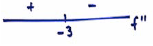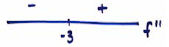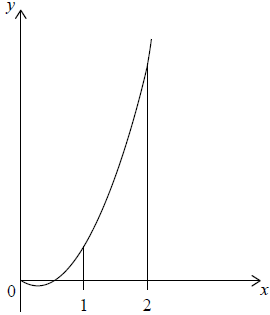# IB Math Analysis & Approaches Question bank-Topic: SL 5.5 integration as anti-differentiation SL Paper 1

## Question

Find  $$\int {\frac{1}{{2x + 3}}} {\rm{d}}x$$ .


a.

Given that $$\int_0^3 {\frac{1}{{2x + 3}}} {\rm{d}}x = \ln \sqrt P$$ , find the value of P.


b.

## Markscheme

$$\int {\frac{1}{{2x + 3}}} {\rm{d}}x = \frac{1}{2}\ln (2x + 3) + C$$  (accept $$\frac{1}{2}\ln |(2x + 3)| + C$$ )    A1A1     N2

[2 marks]

a.

$$\int_0^3 {\frac{1}{{2x + 3}}} {\rm{d}}x = \left[ {\frac{1}{2}\ln (2x + 3)} \right]_0^3$$

evidence of substitution of limits     (M1)

e.g.$$\frac{1}{2}\ln 9 – \frac{1}{2}\ln 3$$

evidence of correctly using $$\ln a – \ln b = \ln \frac{a}{b}$$ (seen anywhere)     (A1)

e.g. $$\frac{1}{2}\ln 3$$

evidence of correctly using $$a\ln b = \ln {b^a}$$ (seen anywhere)     (A1)

e.g. $$\ln \sqrt {\frac{9}{3}}$$

$$P = 3$$ (accept $$\ln \sqrt 3$$ )     A1     N2

[4 marks]

b.

## Question

A function f (x) has derivative f ′(x) = 3x2 + 18x. The graph of f has an x-intercept at x = −1.

Find f (x).


a.

The graph of f has a point of inflexion at x = p. Find p.


b.

Find the values of x for which the graph of f is concave-down.


c.

## Markscheme

evidence of integration       (M1)

eg  $$\int {f’\left( x \right)}$$

correct integration (accept absence of C)       (A1)(A1)

eg  $${x^3} + \frac{{18}}{2}{x^2} + C,\,\,{x^3} + 9{x^2}$$

attempt to substitute x = −1 into their = 0 (must have C)      M1

eg  $${\left( { – 1} \right)^3} + 9{\left( { – 1} \right)^2} + C = 0,\,\, – 1 + 9 + C = 0$$

Note: Award M0 if they substitute into original or differentiated function.

correct working       (A1)

eg  $$8 + C = 0,\,\,\,C = – 8$$

$$f\left( x \right) = {x^3} + 9{x^2} – 8$$      A1 N5

[6 marks]

a.

METHOD 1 (using 2nd derivative)

recognizing that f” = 0 (seen anywhere)      M1

correct expression for f”      (A1)

eg   6x + 18, 6p + 18

correct working      (A1)

6+ 18 = 0

p = −3       A1 N3

METHOD 1 (using 1st derivative)

recognizing the vertex of f′ is needed       (M2)

eg   $$– \frac{b}{{2a}}$$ (must be clear this is for f′)

correct substitution      (A1)

eg   $$\frac{{ – 18}}{{2 \times 3}}$$

p = −3       A1 N3

[4 marks]

b.

valid attempt to use f” (x) to determine concavity      (M1)

eg   f” (x) < 0, f” (−2), f” (−4),  6x + 18 ≤ 0correct working       (A1)

eg   6x + 18 < 0, f” (−2) = 6, f” (−4) = −6f concave down for x < −3 (do not accept ≤ −3)       A1 N2

[3 marks]

c.

## Question

Let $$f\left( x \right) = 6{x^2} – 3x$$. The graph of $$f$$ is shown in the following diagram.Find $$\int {\left( {6{x^2} – 3x} \right){\text{d}}x}$$.


a.

Find the area of the region enclosed by the graph of $$f$$, the x-axis and the lines x = 1 and x = 2 .


b.

## Markscheme

$$2{x^3} – \frac{{3{x^2}}}{2} + c\,\,\,\left( {{\text{accept}}\,\,\frac{{6{x^3}}}{3} – \frac{{3{x^2}}}{2} + c} \right)$$     A1A1 N2

Notes: Award A1A0 for both correct terms if +c is omitted.
Award A1A0 for one correct term eg $$2{x^3} + c$$.
Award A1A0 if both terms are correct, but candidate attempts further working to solve for c.

[2 marks]

a.

substitution of limits or function (A1)

eg  $$\int_1^2 {f\left( x \right)} \,{\text{d}}x,\,\,\left[ {2{x^3} – \frac{{3{x^2}}}{2}} \right]_1^2$$

substituting limits into their integrated function and subtracting     (M1)

eg  $$\frac{{6 \times {2^3}}}{3} – \frac{{3 \times {2^2}}}{2} – \left( {\frac{{6 \times {1^3}}}{3} + \frac{{3 \times {1^2}}}{2}} \right)$$

Note: Award M0 if substituted into original function.

correct working      (A1)

eg  $$\frac{{6 \times 8}}{3} – \frac{{3 \times 4}}{2} – \frac{{6 \times 1}}{3} + \frac{{3 \times 1}}{2},\,\,\left( {16 – 6} \right) – \left( {2 – \frac{3}{2}} \right)$$

$$\frac{{19}}{2}$$     A1 N3

[4 marks]

b.